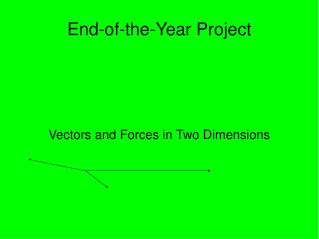DownloadDownload PresentationEnd-of-the-Year Project

# End-of-the-Year Project

Télécharger la présentation## End-of-the-Year Project

- - - - - - - - - - - - - - - - - - - - - - - - - - - E N D - - - - - - - - - - - - - - - - - - - - - - - - - - -
##### Presentation Transcript

1. Vectors and Forces in Two Dimensions End-of-the-Year Project

2. Definitions • Vector: a quantity possessing both magnitude and direction, represented by an arrow the direction of which indicates the direction of the quantity and the length of which is proportional to the magnitude • Force: a dynamic influence that changes a body from a state of rest to one of motion or changes its rate of motion. The magnitude of the force is equal to the product of the mass of the body and its acceleration

3. One Dimension • In one dimension, vectors can be easily added. 10m 6m + = 1m 8m = + Not drawn to scale

4. What's the use? • It may look like just lines, but really it's more than that. Vectors aren't just for distances, but anything with direction and magnitude. For example, there are force vectors and velocity vectors • These can be used to represent forces on an object. 15N 20N Not drawn to scale

5. It's simple in one dimension... • Vector addition is very simple in one dimension, basically just an addition problem. • In two dimensions, it gets more complicated. • In two dimensions, there are a few ways to add vectors • Head-to-tail method • Pythagorean theorem • Trigonometry

6. Head-to-Tail Method • When vectors are drawn perfectly in proportion and you have a ruler, an easy way to add vectors is head-to-tail. (note: this works for any number of vectors at any angle)‏ Scale: 1cm=1m

7. Pythagorean Theorem • The Pythagorean theorem is something we've known for years and has many applications. It can be used to add two vectors that are at right angles to each other a²+b²=c² c=√(a²+b²)‏ 13N 15N Not drawn to scale

8. Trig • Trig is fun! Remember SOH CAH TOA • We can determine the direction of the resultant with trig

9. Trig examples 27N 13N Θ Tan Θ=opp/adj Θ=tan^(-1) (opp/adj)‏ Θ=tan^(-1) (27/13)= Not drawn to scale

10. Components • Components make up vectors that are at angles. • This is basically the opposite of adding vectors to find a resultant SOHCAHTOA 7N 40° Not drawn to scale

11. Inclined planes • One of the uses of finding components is figuring out forces on an object on an inclined plane

12. Inclined planes example Mass is 85kg Fw=mg g=9.8m/s² Fw=(9.8)(85)=833N 34° Fparallel=Fw (sin Θ)‏ Fperpendicular=Fw (cos Θ)‏ Fparallel=(833)(sin 34°)=440.7 Fperpendicular=(833)(cos 34°)=-706.9 Not drawn to scale

13. In conclusion... • We always ask “how does this apply to life?” about math... • solving seemingly endless triangles in the math book may seem useless...well it is unless you realize what it actually means and what its connection is to the study of motion and forces.